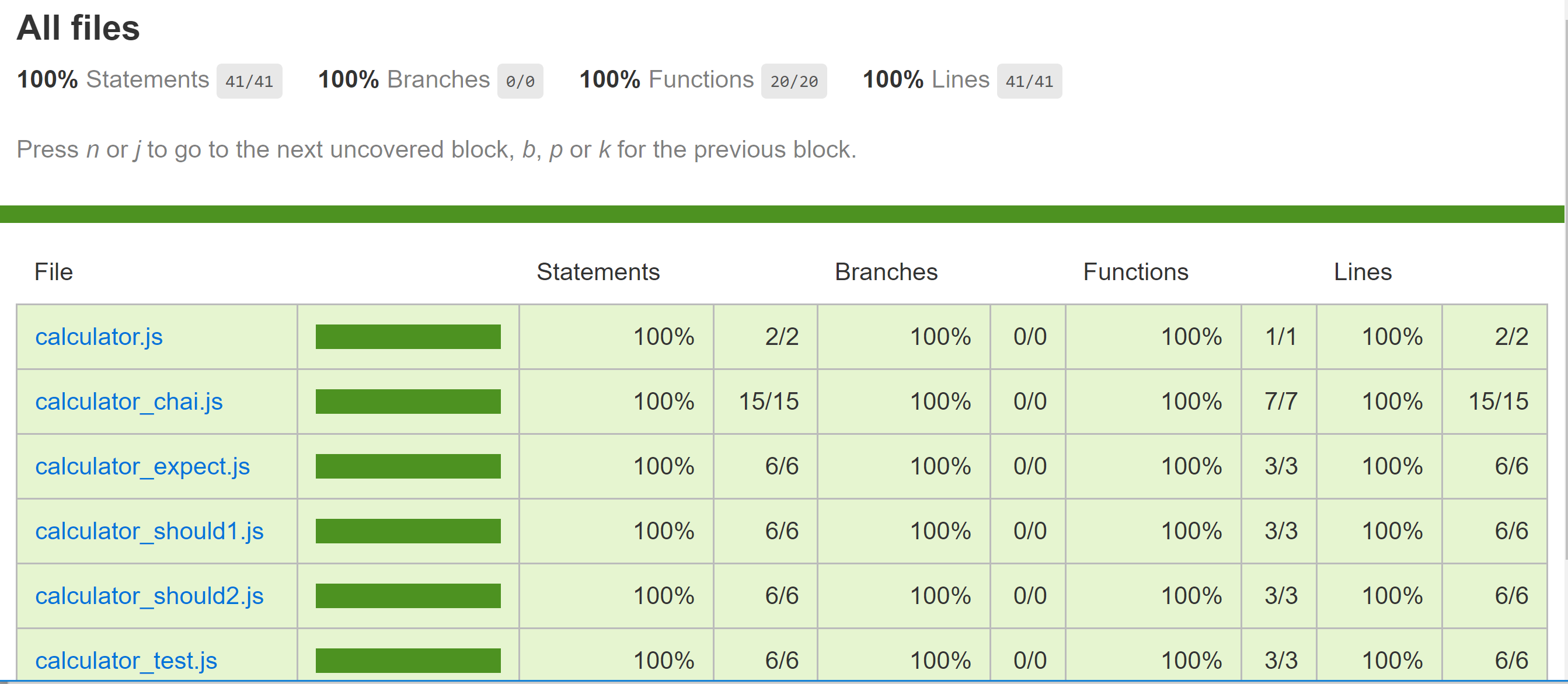## 8 Node.js 单元测试

### 8.1 单元测试

#### 8.1.1 引子

``````function add(a,b) {
return a+b;
}
``````

``````var s = add(1,2);
if (s == 3) {
console.log('恭喜你，加法测试成功了');
} else {
console.error('哎呦，加法测试出错了啊');
}
``````

``````var assert = require('assert');
describe('Calculator', function() {
describe('#add()', function() {
it('should get 3 when 1 add 2', function() {
assert.equal(3, add(1,2));
});
});
});
``````

mocha 在使用的时候，可以选择全局安装的模式，也可以安装为当前项目的开发依赖包（即在安装的时候使用`--save-dev`参数），不过每个项目中使用的版本可能会略有差异，并且部分 IDE 对于全局安装的支持并不是很好，所以推荐安装为当前项目的开发依赖。

``````var assert = require('assert');
var calculator = require('./calculator');

describe('Calculator', function() {
describe('#add()', function() {
it('should get 3 when 1 add 2', function() {
assert.equal(3, calculator.add(1,2));
});
});
});
``````

``````e:\kuaipan\code\node\myapp\chapter7\src\test\mocha>node calculator_test.js
e:\kuaipan\code\node\myapp\chapter7\src\test\mocha\calculator_test.js:4
describe('Calculator', function() {
^
ReferenceError: describe is not defined
at Object.<anonymous> (e:\kuaipan\code\node\myapp\chapter7\src\test\mocha\calculator_test.js:4:1)
at Module._compile (module.js:456:26)
at Object.Module._extensions..js (module.js:474:10)
at Module.load (module.js:356:32)
at Function.Module._load (module.js:312:12)
at Function.Module.runMain (module.js:497:10)
at startup (node.js:119:16)
at node.js:929:3
``````

``````e:\kuaipan\code\node\myapp\chapter7\src\test\mocha>mocha calculator_test.js
Calculator
#add()
√ should get 3 when 1 add 2

1 passing (7ms)
``````

#### 8.1.2 断言

``````var should = require('should');

(calculator.add(1,2)).should.be.exactly(3).and.be.a.Number();
``````

``````var should = require('should/as-function');

should(calculator.add(1,2)).be.exactly(3).and.be.a.Number();
``````

2.expect.js

``````var expect = require('expect.js');
expect(calculator.add(1,2)).to.be(3);
``````

3.chai
chai 将前面提到的assert should expect融合到了一起，你仅仅需要使用 chai 这一个包就能享用以上三者的功能，所以前面讲到的三种判断在chai中是这么实现的：

``````var chai = require('chai');

var assert = chai.assert;//use assert
assert.equal(3, calculator.add(1,2));

chai.should();//use should
(calculator.add(1,2)).should.be.equal(3).and.be.a('number');

var expect = chai.expect;//use expect
expect(calculator.add(1,2)).to.equal(3);
``````

``````to be been is that which and has have with at of same
``````

#### 8.1.3 HTTP 测试

HTTP请求和本地调用从流程是有很多不一样的地方的：当中隔着一个网络层，请求数据以 HTTP 报文的格式在网络上进行传输，服务器端解析 HTTP 报文内容后再调用本地 API 进行处理，然后再将处理结果通过 HTTP 报文返回给调用者。supertest 这个工具可以很好得在 mocha 中处理 HTTP 请求断言。

``````exports.doAdd = function(req, res) {
var _body = req.body;
var a = parseInt(_body.a, 10);
if (isNaN(a)) {
return res.send({code:1,msg:'a值非法'});
}
var b = parseInt(_body.b, 10);
if (isNaN(b)) {
return res.send({code:2,msg:'b值非法'});
}
res.send({code:0,data:a+b});
};
``````

``````var request = require('supertest');
var app = require('../../app');

describe('POST /calculator/add', function() {
it('respond with json', function(done) {
request(app)
.post('/calculator/add')
.send({a: 1,b:2})
.expect(200, {
code:0,data:3
},done);
});
});
``````

``````var request = require('supertest');
var app = require('../../app');
var cookie = exports.cookie = '';

before(function(done) {
request(app)
.post('/user/login')
.send({username:'admin', password:'admin'})
.expect(200,{code:0})
.end(function(err,res) {
if (err) {
return done(err);
}
var header = res.header;
var setCookieArray = header['set-cookie'];

for (var i=0,len=setCookieArray.length;i<len;i++) {
var value = setCookieArray[i];
var result = value.match(/^express_chapter7=([a-zA-Z0-9%\.\-_]+);\s/);
if (result && result.length > 1) {
exports.cookie = cookie = result;
break;
}
}
if (!cookie) {
return done(new Error('查找cookie失败'));
}
done();
});
});
``````

``````var request = require('supertest');
var app = require('../../app');
var before = require('./login_before');

describe('Backend',function() {

it('first test',function(done) {
request(app)
.get('/user/admin')
.set('Cookie','express_chapter7=' + before.cookie)
.expect(200,/<title>admin<\/title>/,done);
});
});
``````

### 8.2 测试覆盖率

`"coverage": "nyc report --reporter=html"`